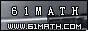Today is . WELCOME: huohai3983/44663  Home | Reg Login | All user | setHomePage | BookmarkHome | Math Test Online | Addition | Subtraction | Multiplication | Division | Time | Worksheet(JPG/PDF) | CAI | Flash Games |Recommendation articleArticle searchHome--maths--Second Grade--Subtracting Two Digit Numbers without Renaming

Subtracting Two Digit Numbers without Renaming

Subtracting Two Digit Numbers without Renaming

How to subtract two-digit numbers (for example 67 - 45).

• Place one number above the other so the tens' place digits and ones' place digits are lined up. Draw a line under the bottom number.
```67
45
```
• Subtract the bottom ones' place digit (5) from the top ones' place digit (7) and place the difference (2) below the line in the ones' place column.
```67
45
2
```
• Subtract the bottom tens' place digit (4) from the top tens' place digit (6). Place the difference (2) below the line in the tens' place column.
```67
45
22```

#### Find the Difference Between the two Numbers.

 -     =

2006-12-14 22:43:19
Viewed 4069 times CloseGreatmathsites

### Translate

 Thank you to online math channels' Mathematics Department for hosting these lessons. Copyright © 2006 - 2008 GZtrain Inc. All Rights Reserved